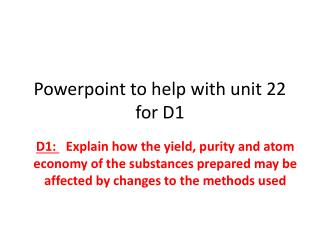DownloadDownload PresentationPowerpoint to help with unit 22 for D1

# Powerpoint to help with unit 22 for D1

Download Presentation## Powerpoint to help with unit 22 for D1

- - - - - - - - - - - - - - - - - - - - - - - - - - - E N D - - - - - - - - - - - - - - - - - - - - - - - - - - -
##### Presentation Transcript

1. Powerpoint to help with unit 22 for D1 D1: Explain how the yield, purity and atom economy of the substances prepared may be affected by changes to the methods used

2. For the distinction task you need to: • Suggest at least three ways in which yield and purity can be changed by adapting the methods used for each preparation (aspirin and copper (II) sulfate-5-water). • Give the balanced equation and the atom economy of each preparation used in the lab. • Give one alternative preparation for each substance which would result in a different atom economy from the ones in the methods used. • Calculate the atom economy of each of these alternative preparations, and compare them with the atom economy for the procedure used in the college lab

3. 1. Suggest at least three ways in which yield and purity can be changed by adapting the methods used for each preparation (aspirin and copper (II) sulfate-5-water). • Read through the method for making copper (II) sulfate-5-water and then the method for making aspirin. • For each method find THREE ways/parts of the method that could have reduced the yield and/or purity of the products. • Then discuss how you could minimise or deal with these ways/parts of the method that could have reduced the yield and/or impurity of the products.

4. Things to look for in the methods when identifying Ways/parts of methods that could reduce yield/purity: • Did you heat the chemicals enough or over heat them? • Did you have to add the chemicals bit by bit or in one go? • Did you evaporate the chemicals properly? • Did you filter and then collect ALL of the desired product? • Did you give the chemicals enough time to cool? • Did you ‘wash’ the chemicals thoroughly during filtration? • If an ice bath was used did you leave the chemicals in there long enough to cool down? • If you carried out a distillation did you successfully separate out the desirable products? • If you carried out a reflux did you leave enough time for the reaction to complete?

5. 2. Give the balanced equation and the atom economy of each preparation used in the lab • Copy and paste your balanced equation and your calculation for atom economy of making copper (II) sulfate-5-water that you have used for the P2 section of the assignment • Copy and paste your balanced equation and your calculation for atom economy of making aspirin that you have used for the P2 section of the assignment

6. 3. Give one alternative preparation for each substance which would result in a different atom economy from the ones in the methods used • Alternative method to making copper (II) sulfate-5-water that you could consider: • Using copper hydroxide INSTEAD OF copper oxide. • This would have the chemical equation: Cu(OH)2+ H2SO4 CuSO4 + 2H2O • You would use a similar method that you did for P1, adding equal amounts of copper hydroxide to sulfuric acid, heating, evaporating and filtering (you will need to type up a proper method)

7. Alternative method to making aspirin that you could consider: • Using acetyl chloride INSTEAD OF ethanoic anhydride. • This would have the chemical equation: • You would use a similar method to the one used for making aspirin for P1 but instead of using ethanoic anhydride you use acetyl chloride (you will need to type up a proper method) Acetyl chloride 2-hydroxybenzoic acid Aspirin

8. 4. Calculate the atom economy of each of these alternative preparations, and compare them with the atom economy for the procedure used in the college lab Don’t forget the formula used to calculate atom economy: % atom economy = __ molar mass desirable product__ X 100 molar mass of all reactants Also you must compare the calculated atom economies of the alternative methods to the actual methods that you used in the practicals (which was calculated in P2 part of assignment)

9. Cu(OH)2+ H2SO4 CuSO4 + 2H2O To calculate the atom economy: % atom economy = __ molar mass desirable product__ X 100 molar mass of all reactants Molar mass of ALL reactants : (Cu(OH)2 and H2SO4) = 195.5 Molar mass of desirable product: (CuSO4) = 160 • Calculating atom economy of alternative method to making copper (II) sulfate

10. Calculating atom economy of alternative method to making aspirin To calculate the atom economy: % atom economy = __ molar mass desirable product__ X 100 molar mass of all reactants Molar mass of ALL reactants : C6H4(OH)COOH + C2H3OCl = 216.5 Molar mass of desirable product: (C6H4COOHOCOCH3) = 180